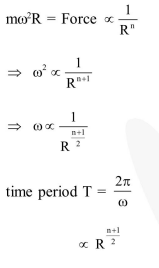# A particle is movingQuestion:

A particle is moving with a uniform speed in a circular orbit of radius $\mathrm{R}$ in a central force inversely proportional to the $n^{\text {th }}$ power of $R$. If the period of rotation of the particle is $T$, then,

1. $T \propto R^{\frac{n}{2}+1}$

2. $T \propto R^{(\mathrm{n}+1) / 2}$

3. $\mathrm{T} \propto \mathrm{R}^{\mathrm{n} / 2}$

4. $T \propto R^{3 / 2}$ for any $n$

Correct Option: , 2

Solution: